﻿ Solving Multi Criteria Decision Aiding (MCDA) Problems Using SpreadsheetsAlgorithms Research

p-ISSN: 2324-9978    e-ISSN: 2324-996X

2013;  2(2): 29-42

doi:10.5923/j.algorithms.20130202.01

### Solving Multi Criteria Decision Aiding (MCDA) Problems Using Spreadsheets

T. Ganesh, PRS Reddy

Department of Statistics, S.V. University, Tirupati, India

Correspondence to: T. Ganesh, Department of Statistics, S.V. University, Tirupati, India.
 Email: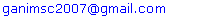Abstract

In Managerial Decision making, the problem environment will be encircled by a set of alternatives for set of criteria. The main objective is to choose the best alternative under each criterion. In this contest, the Decision Maker (DM) plays an important role in solving the hard/complex problems. This type of scenario gives raise to the concept of MCDA. In this paper, we made an attempt to provide some algorithms which are user-friendly. In this paper, we have provided some algorithms which supports in computing the concordance and discordance indices.

Keywords: Multi Criteria, Concordance, Discordance, Outranking Index

Cite this paper: T. Ganesh, PRS Reddy, Solving Multi Criteria Decision Aiding (MCDA) Problems Using Spreadsheets, Algorithms Research , Vol. 2 No. 2, 2013, pp. 29-42. doi: 10.5923/j.algorithms.20130202.01.

### 1. Introduction

In any environment, the main objective is to provide a set of best alternatives for given criteria. The decision maker provides some necessary and basic information about each criterion and the alternatives that helps in identifying the relation between them. The problems of this kind can be dealt with Multi Criteria Decision Making or Multi Criteria Decision Aid (MCDA) techniques.
The main aim of MCDA is to account for several views and provide some tools for the Decision Maker (DM) in solving complex decision problems. The trade-off between the criteria and DM’s preferences lies in providing compromise solutions. In each and every problem or situation, the DM, Stakeholder and Analyst play an important role.
DM is a person, who has a great impact in evaluating the situation, expressing preferences, considering solutions and approving the final result. Stakeholders are members involved in decision situation and interested in finding a solution for the problem. For the situation considered, the Analyst is responsible in recognizing the consequences and selecting an appropriate decision aiding method/tool for the construction of decision models.
In every MCDA problem environment, each criterion will be embedded with a set of alternatives out of which one alternative will act as the best for that particular criterion. These set of alternatives will be finite if a proper definition about all the members is given, otherwise infinite. If the number and content of alternatives are fixed and cannot be varied during the decision aiding process, then this nature is said to be stable otherwise volatile. At the final stage of the decision aiding process, if we come across a single best alternative which excludes the possibility of choosing any other alternative, it is referred as Comprehensive and if we opt for a combination of alternatives, it is fragmented. . In brief, the alternatives are estimated on a set of criteria. The criterion defines the feature and some properties of the set of alternatives.
Notations
xi : ith alternative (i=1,…,m)
X : Set of alternatives
gj : jth criterion (j=1,…,n)
G : set of criteria
Qj : jth Indifference thresholds
Pj : jth Preference thresholds
Wj : jth Weights
Vj : jth Veto thresholds
λ : Cutting level
bq : qth boundary alternative (q = 1,…,s)
B : set of boundary alternatives (b1, b2, …, bq)
lq : qth boundary class
Cj (xi, bq) and Dj (xi, bq) : partial concordance and partial discordance of the xi and bq
Cj (bq, xi) and Dj (bq, xi) : partial concordance and partial discordance of the bq and xi
C (xi, bq) and C (bq, xi) : overall concordance indices
Sj (xi, bq): outranking index for xi and bq
Sj (bq, xi): outranking index for bq and xi
Cq : qth category
P : strict preference
Q : weak preference
I : indifference
J : incomparability
The entire MCDA problem will be expressed in terms of relations existing between the alternatives and criteria. We brief out each and every relation and the nomenclature for it.

#### 1.1. Relations

• The indifference relation between two alternatives xi and xr, denoted as xiIxr, means that the two alternatives xi and xr are equally preferable or equally important to the DM. This relation is reflexive and symmetric.
• The strict preference is a relation of xi over xr, denoted as xiPxr, which gives the meaning that xi is better than the xr for the DM. It is asymmetric and non-reflexive.
• The weak preference is a relation which hesitates to make a specific judgment about the preference or indifference between xi and xr, denoted by xiQxr. It is also asymmetric and non-reflexive.
• If xi is not in any of the above mentioned relations with xr, then it is referred to as incomparability relation, denoted by xiJxr. This relation is symmetric and non-reflexive.
• The outranking relation is denoted as xiSxr. It defines the situation in which the preference (strong- xiPxr or weak- xiQxr) or indifference relation (xiIxr) is true or not.
In order to observe a specific type of relation between alternative and criterion, there is a need to compute some indices such as partial concordance, discordance and outranking indices. over the years, many methodologies were developed of which the most familiar method is the Outranking Methodology. In outranking methodology, we have considered ELECTRE TRI method and for this we have developed spreadsheet algorithms, which support the analyst to analyze and to provide a better decision making. First we review some literature confining to ELECTRE TRI method and then a detailed algorithmic approach is given along with the results.

### 2. Outranking Methodology

In MCDA, the outranking methodology comes under the framework of classification problems. Basing on the same criterion, the methodology allows comparing the pairs of alternatives by considering indifference, preference and veto thresholds. This helps in determining the indifference, preference to one over the other and incomparable relation between alternatives. The seminal work on this methodology was proposed by B. Roy (1965). He developed some mathematical structures about the ELECTRE family which help in choosing the best alternative from the set of alternatives. In recent years, many state of art surveys were conducted and reported on the development of the MCDA methodologies by M.Bruen and L. Maystre (2000), B.Roy and J. Figueira (2002), J. Martel and B. Matarezzo (2005), J. Figueira, V. Mousseau and B.Roy (2005).
B. Roy (1977, 1981) proposed the Trichotomic segmentation outranking based classification method for sorting problems with three classes. Later, this method was extended to an arbitrary number of classes in N-TOMIC by R. Massagliaet (1991) and few ELECTRE methods by V. Mousseau et al (1998) and W. Yu (1992).

#### 2.1. ELECTRE TRI Method

ELECTRE method helps to identify the outranking relations between pairs of alternatives for each criterion. In classification problems, a given set of alternatives X with a set of criteria G are to be assigned into a set of ordered classes L by the predefined set of boundary alternatives B. Each class is considered by two (upper and lower) boundary alternatives. The upper bound bq of the class lq-1 is the lower bound of the class lq (q=1,…,s). Changing the least one criterion moves the boundary alternative to the neighbouring class.
For solving the classification problem the method estimates the outranking relation for each alternative xi ϵ X (i=1,…,m) which is to be classified and each boundary alternative bq between classes lq-1 and lq by calculating the outranking index. If lq is preferred to the lower boundary alternative lq-1 of the class, we assign the alternative xi to the class lq and the upper boundary alternative bq of the class is preferred to this alternative.
For calculating the outranking index, the DM should give the information about
(i) the set of alternatives to be classified
(ii) the set of criteria on which alternatives are evaluated with a scale of quantitative values for each criterion.
(iii) the number of classes as well as their order according to preference.
(iv) the upper and lower boundary alternatives for each class lq
For each criterion gj (j=1,…,n), the ELECTRE TRI method requires to define the preference pj(.), indifference qj(.), veto vj(.) thresholds as well as weights wj and cutting level λ (should lie between 0.5 and 1).
(a) the preference pj(.) threshold indicates the smallest difference between two alternatives on the criterion gj, that is one alternative is preferred to the other.
(b) the indifference qj(.) threshold indicates the largest difference between two alternatives on the criterion gj.
(c) the veto vj(.) threshold indicates the smallest difference between the alternatives on the criterion gj, that says incomparability of these two alternatives.
(d) All the above three thresholds should satisfy the constraint, vj (.) > pj (.) > qj (.)
(e) the weight wj indicates the relative importance of criterion when compare to the other criterion in terms of votes.
(f) the cutting level λ shows the smallest value of the outranking index, which is sufficient for considering an outranking situation between two alternatives.
The outranking relation is verified by two conditions; concordance and discordance, with respect to the thresholds, weights and cutting level λ. Concordance requires preference of the alternative xi over the boundary alternative bq on the majority of criteria. Discordance demands the absence of strong opposition to the first condition in the majority of criteria. We need to compute two partial indices for each criterion, that is partial concordance Cj (xi, bq) and Cj (bq, xi) and partial discordance Dj (xi, bq) and Dj (bq, xi). The above partial indices help in computing the outranking indices Sj (xi, bq) and Sj (bq, xi). Using a specific cutting level λ, a comparison of outranking indices is possible and turns to two types of assignment procedures namely pessimistic and optimistic.
The pessimistic procedure starts with the comparison of an alternative to the lower bound of the highest class and the optimistic procedure starts with the comparison of an alternative to the upper bound to the lowest class. In section 3, we describe the mathematical structures of outranking indices and assignment procedures.

### 3. Algorithm of the ELECTRE TRI Method

The ELECTRE TRI method has been divided into two parts; part I is to compute the outranking indices and to identify the relations between the alternatives and criteria and in part II, using the obtained outranking relation and cutting level λ, we provide the final result for the MCDA problem.
Part I: To construct the outranking relation xi S bq for each alternative xi to be classified and each boundary alternative bq.
1. Calculate the partial concordance indices Cj (xi, bq) and Cj (bq, xi) for each criteria gj according to the increasing direction of preferences. The partial concordance index Cj (xi, bq) is as follows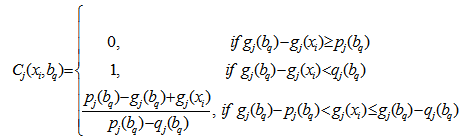The partial concordance index Cj (bq, xi) is as follows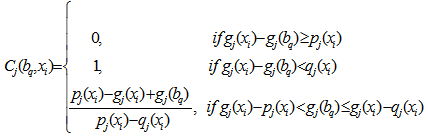2. To find the overall concordance indices C (xi, bq) and C (bq, xi) as an aggregation of partial concordance indices.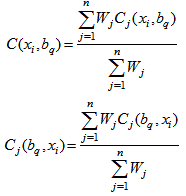3. Calculate partial discordance indices Dj (xi, bq) and Dj (bq, xi) for each criteria gj. We compute the partial discordance index Dj (xi, bq) according to the increasing direction of preference.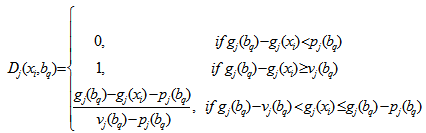The partial discordance index Dj (xi, bq) is as follows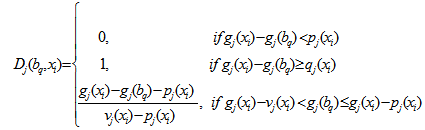4. Calculate the outranking indices S(xi, bq) and S(bq, xi), that shows outranking creditability. The creditability index of xi over bq assuming S(xi, bq) ϵ[0,1] as follows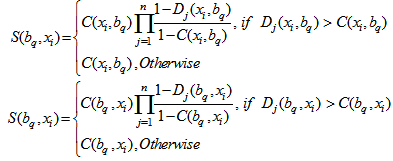5. The value of outranking indices is compared to the cutting level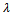, which is defined by the DM and lies in the interval[0.5, 1].
• If S(xi, bq)and S(bq, xi)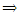xiIbq, then the alternative xi and bq are indifferent.
• If S(xi, bq)and S(bq, xi) <xiPbq or xiQbq, then the alternative xi is strongly or weakly preferred to the boundary alternative bq.
• If S(xi, bq) <and S(bq, xi)bqPxi or bqQxi, then the boundary alternative bq is strongly or weakly to xi.
• If S(xi, bq) <and S(bq, xi) <xiJbq, then the alternative xi and bq are incomparable.
Part II:
On using the computed outranking indices in Part I, the DM has an option to choose either an optimistic procedure or a pessimistic procedure or both. After choosing an alternative procedure, the comparison of outranking indices for each pair of alternative xi will be classified using each boundary alternative to the cutting level.

#### 3.1. The Pessimistic Procedure

In this procedure the comparison will start from alternative xi to the lower bound bq-1 of the highest class lq (q=s,…,1) and continues in decreasing order until, a lower bound bq-1 is found, that is xiSbq-1, and for estimating the outranking relation we calculate S(xi , bq-1). Once the outranking relation is obtained, we calculate outranking index between xi and bq. We assign the alternative xi to the lq if S(xi, bq-1)and S(xi, bq) <.
1. Compare xi successively to bq for q= s,s-1,…,0
2. bq being the first bound such that xiSbq, assign xi to category Cq+1 (xiCq+1)
In other words, the above procedure can also be expressed as follows; bq-1 and bq are upper and lower bound of category Cq, the pessimistic procedure assigns alternative xi to the highest category Cq such that xi Sbq-1. When using this procedure with=1, an alternative xi can be assign to category Cq only if gj(xi) equals or exceeds gj(bq-1) for each criterion. Whendecreases the pessimistic characters of this rule is weakened.

#### 3.2. The Optimistic Procedure

Here, we begin to compare the alternative xi to the upper bound bq of the lowest class lq (q=1,…,s) and proceed in increasing order until we find such a upper bound bq that has strict preferences over the alternatives xi, then we calculate S(xi, bq-1) and assign that alternative to the class lq if S(xi, bq-1)and S(xi, bq) <.
1. Compare xi successively to bq for q=1,…,s.
2. bq being the first bound such that bqPxi, assign xi to Cq (xiCq)
The optimistic procedure assign to xi to the lowest category Cq for which the upper bound bq is preferred to xi. When using this procedure with= 1, an alternative xi can be assigned to category Cq when gj(bq) exceeds gj(xi) at least for one criterion. Whendecreases the optimistic character of this rule is weakened.

#### 3.3. Comparison of Two Assignment Procedures

Let us suppose that an alternative xi is assigned to Cq and Cr by the pessimistic and optimistic procedures, if the following conditions holds good
Cq is lower or equal to Cr (q ≤ r)
Cq > Cr, when xiJbF for every F, rF < q.
More specifically when the evaluation of an alternative are between the two boundary alternatives of a category on each criterion, then both procedures assign this alternative to this criterion. xi divergence exists among the results of the two assignment procedures only when an alternative is incomparable to one or several bq, in such case the pessimistic rule assigns the alternative to lower category than the optimistic.
Here, we demonstrate a spreadsheet algorithm for the ELECTRE TRI method using a numerical illustration. We have programmed two algorithms, of which the first one helps in finding the values of partial concordance and discordance along with the outranking index between xi and bq and the second algorithm provides solution for bq and xi.
Algorithm 3.1
Step 1: Enter the criteria values along with alternatives in ‘mxn’ design.
Step 2: Enter threshold values in a separate row below to the mxn design.
Step 3: To compute the partial concordance between ith criteria and jth alternative Cj(xi, bq) the following ‘NESTED IF ( )’ condition has been used
=IF ((B6-B10)>=B2, 0, IF ((B6-B9)Step 4: Repeat Step 3 for finding the left out concordance values.
Step 5: The overall concordance of two alternatives C(xi, bq) can be obtained using
‘SUMPRODUCT()’function =SUMPRODUCT (H2:L2,B11:F11)/SUM(B11:F11)
Step 6: To compute the partial discordance between ith criteria and jth alternative Dj(xi, bq), the following ‘NESTED IF ( )’ condition has been used
=IF((B20-B24)=B16,1,((B20-B16-B24)/(B26-B24))))
Step 7: To compute the out ranking index between ith criteria and jth alternative S(xi, bq) the following ‘IF ( )’ condition has been used
=IF(H16>\$S\$2,(\$S\$2*(1-H16)/(1-\$S\$2)),\$S\$2)
Algorithm 3.2
Step 1: Enter the criteria values along with alternatives in ‘mxn’ design.
Step 2: Enter threshold values in a separate row below to the mxn design.
Step 3: To compute the partial concordance between ith criteria and jth alternative Cj(bq , xi) the following ‘NESTED IF ( )’ condition has been used
=IF((B6+B10)<=B2,0,IF((B6+B9)>B2,1,((B6-B2+B10)/(B10-B9))))
Step 4: Repeat Step 3 for finding the left out concordance values.
Step 5: The overall concordance of two alternatives
C(bq , xi) can be obtained using ‘SUMPRODUCT()’function =SUMPRODUCT(N2:R2,B11:F11)/SUM(B11:F11))
Step 6: To compute the partial discordance between ith criteria and jth alternative Dj(bq , xi), the following ‘NESTED IF ( )’ condition has been used
=IF((B16-B20)=B26,1,((B16-B20-B24)/(B26-B24))))
Step 7: To compute the out ranking index between ith criteria and jth alternative S(bq , xi) the following ‘IF ( )’ condition has been used
=IF(H16>\$T\$2,(\$T\$2*(1-H16)/(1-\$T\$2)),\$T\$2)

### 4. Numerical Illustrations

Let us consider an MCDA problem which has five criteria and three alternatives for each criterion. The table below gives the boundary alternatives b1 and b2 and various thresholds given by the decision maker (DM).

#### 4.1. EXAMPLE 1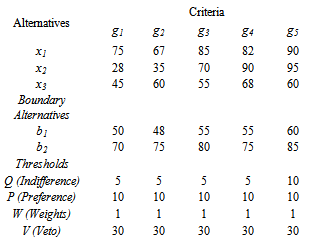Now, using the algorithm 3.1 and 3.2, the following values are computed. Along with the partial concordance and discordance, the overall concordance is also reported in the tables 1, 2, 3 and 4.
 Table 1. Partial concordance of Cj(xi, bq)
 Partial Concordance of Cj(xi, bq) g1 g2 g3 g4 g5 Cj(x1,b1) 1 1 1 1 1 Cj(x2,b1) 0 0 1 1 1 Cj(x3,b1) 1 1 1 1 1 Cj(x1,b2) 1 0.4 1 1 1 Cj(x2,b2) 0 0 0 1 1 Cj(x3,b2) 0 0 0 0.6 0
 Table 2. Partial concordance and overall concordance
 Partial Concordance for Cj(bq, xi) Overall Concordance g1 g2 g3 g4 g5 C(xi,b) C(b,xi) Cj(b1,x1) 0 0 0 0 0 1 0 Cj(b1,x1) 1 1 0 0 0 0.6 0.4 Cj(b1,x3) 1 0 1 0 1 1 0.6 Cj(b2,x1) 1 1 1 0.6 1 0.88 0.92 Cj(b2,x1) 1 1 1 0 0 0.4 0.6 Cj(b2,x3) 1 1 1 1 1 0.12 1
 Table 3. Partial discordance for Dj(bq, xi)
 Partial discordance for Dj(bq, xi) g1 g2 g3 g4 g5 Dj(b1,x1) 0.75 0.45 1 0.85 1 Dj(b1,x2) 0 0 0.25 1 1 Dj(b1,x3) 0 0.1 0 0.15 0 Dj(b2,x1) 0 0 0 0 0 Dj(b2,x2) 0 0 0 0.25 0 Dj(b2,x3) 0 0 0 0 0
 Table 4. Partial discordance for Dj(xi, bq)
 Partial discordance for Dj(xi, bq) g1 g2 g3 g4 g5 Dj(x1,b1) 0 0 0 0 0 Dj(x2,b1) 0.6 0.15 0 0 0 Dj(x3,b1) 0 0 0 0 0 Dj(x1,b2) 0 0 0 0 0 Dj(x2,b2) 1 1 0 0 0 Dj(x3,b2) 0.75 0.25 0.75 0 0.75
On the basis of the above four tables, we have calculated the outranking indices for both S(bq, xi) and S(xi, bq)
 Table 5. Outranking indices for S(xi, bq)
 Outranking indices for S(xi, bq) g1 g2 g3 g4 g5 S(x1, b1) 0 0 0 0 0 S(x2, b1) 0.267 0.4 0.4 0.4 0.4 S(x3, b1) 0.6 0.6 0.6 0.6 0.6 S(x1, b2) 0.92 0.92 0.92 0.92 0.92 S(x2, b2) 0 0 0.6 0.6 0.6 S(x3, b2) 1 1 1 1 1
 Table 6. Outranking indices for S(xi, bq)
 Outranking indices for S(bq, xi) g1 g2 g3 g4 g5 S(b1, x1) 1 1 1 1 1 S(b1, x2) 0.6 0.6 0.6 0.6 0.6 S(b1, x3) 1 1 1 1 1 S(b2, x1) 0.88 0.88 0.88 0.88 0.88 S(b2, x2) 0 0 0.4 0.4 0.4 S(b2, x3) 0.034 0.102 0.034 0.12 0.034
The table 7 gives a picture about the outranking relation between the criteria and alternatives.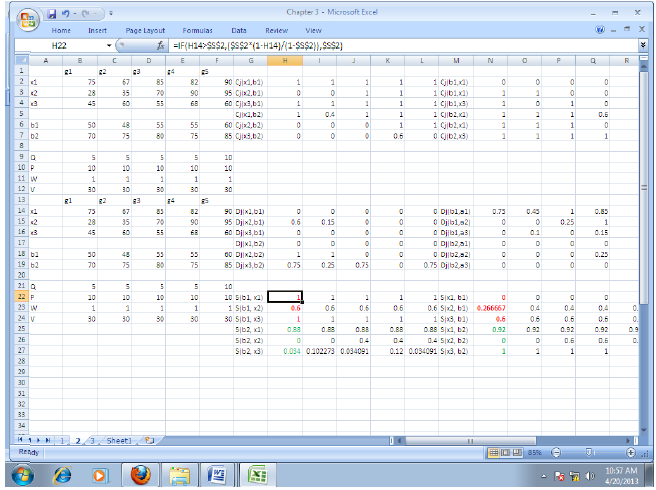Table 7. Outranking relation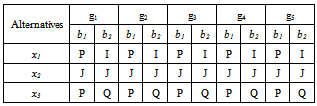After obtaining the Outranking indices, the decision maker will decide the cutting level λ. Using this, the comparison will be done between the alternatives and criteria. Here, the cutting level λ is taken as 0.75. In this problem, we have defined two boundary alternatives that is b1 and b2. First let us consider the boundary alternative b1 with three alternatives for g1. The values of the indices S (x1, b1) and S (b1, x1) hold the relation P (strictly preference), since S (x1, b1) > λ and S (b1, x1) < λ. In similar fashion, if we compare S (x2, b1) and S (b1, x2) with λ, an Indifference relation (I) is noticed since these two relations are less than λ. Finally, on comparing S (x3, b1) and S (b1, x3) with λ, it is observed that S (x3, b1) < λ and S (b1, x3) > λ, which means that the outranking relation is of weak preference (Q). So here, we made an attempt to demonstrate all sorts of relations between the criteria and boundary alternatives using an MCDA problem. Further, let us consider another boundary alternative b2 for three alternatives to explain and observe what sort of relations exists between them. It is observed that S (x1, b2) and S (b2, x1) > λ, then the outranking relation is Incomparable (I). Similarly, if we compare S (x2, b2) and S (b2, x2) with λ, the two relations are less than λ indicating that outranking relation is Indifference (I). Again on comparing S (x3, b2) and S (b2, x3) with λ, it is observed that S (x3, b2) < λ and S (b2, x3) > λ, the outranking relation is weak preference (Q). Once the outranking relations are identified, the DM will choose any one of the assignment procedures. Here, we have briefly discussed both the procedures for the same problem.
Results of ELECTRE TRI Pessimistic procedure:
x1 is assigned to C3 because x1Sb3 does not hold but x1Sb2 holds
x2 is assigned to C1 because x2Sb3, x2Sb2 and x2Sb2 do not hold but x2Sb0 holds.
x3 is assigned to C1 because x3Sb3 and x3Sb2 does not hold but x3Sb1 holds.
Results of ELECTRE TRI Optimistic procedure:
x1 is assigned to C3 because b0Px1, b1Px1 and b2Px1 do not holds but b3Px1 holds
x2 is assigned to C3 because b0Px2, b1Px2 and b2Px2 do not holds but b3Px2 holds.
x3 is assigned to C2 because boPx3, b1Px3 does not holds but b2Px2 holds.
It is observed that x2 is assigned to C3 by the optimistic procedure and C1 by the pessimistic procedure. This shows that, x2 is incomparable to both the boundary alternatives b1 and b2 which in turn gives the meaning that in spite of different priorities, x2 alternative is the preferable one in each and every criterion. Similar kind of interpretation can be given for the remaining criteria.g2, g3, g4 and g5.

#### 4.2. EXAMPLE 2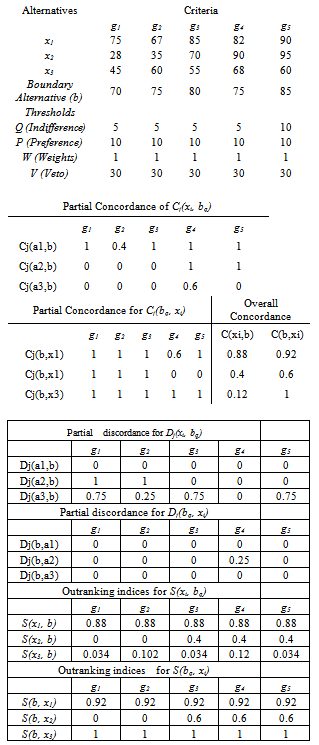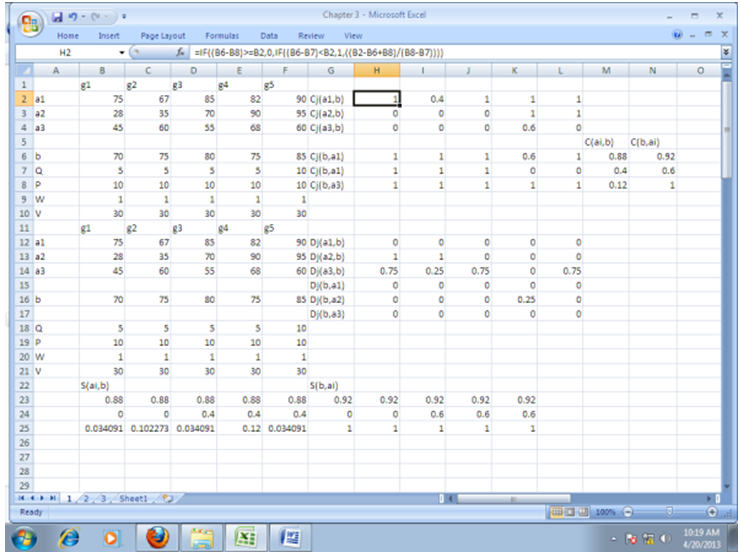### 5. Conclusions

In MCDA problem, the outranking methodology of ELECTRE TRI method provides a compromise solution. In this paper, we have focused on the usage of spreadsheet procedures for the MCDA problem with ELECTRE TRI method. Further, we have considered two boundary alternatives and highlighted the importance of them. Finally, with the help of the outranking indices and relations, we have interpreted that the alternative x2 is considered to be the best among three alternatives for every criterion. We have considered an MCDA problem which explains all sorts of outranking relations between the boundary alternatives and criteria. The algorithms are user friendly and flexible in handling the MCDA problem with ‘n’ boundary alternatives. The algorithm proposed is a user friendly one and allows user to handle the complex dimensioned MCDA problems very simply using the defined macro. Even though, separate software exists for ELECTRE TRI method, but it is not that easy to access and understand. However, this macro allow user to define the preferences, weights and thresholds. This macro is so handy and with a limited nested – if functions one can easily understand the anatomy of the ELECTRE TRI method.

### ACKNOWLEDGEMENTS

The first author would like to acknowledge UGC-BSR for their financial support.

### Macro Used for Solving MCDA problems

Ganesh()
'
' Ganesh Macro
'
' Keyboard Shortcut: Ctrl+Shift+G
'
Range("H2").Select
ActiveCell.FormulaR1C1 = _
"=IF((RC[-6]-RC[-6])>=RC[-6],0,IF((RC[-6]-RC[-6]) Range("H2").Select
Selection.AutoFill Destination:=Range("H2:L2"), Type:=xlFillDefault
Range("H2:L2").Select
Range("H3").Select
ActiveCell.FormulaR1C1 = _
"=IF((RC[-6]-RC[-6])>=RC[-6],0,IF((RC[-6]-RC[-6]) Range("H3").Select
Selection.AutoFill Destination:=Range("H3:L3"), Type:=xlFillDefault
Range("H3:L3").Select
Range("H4").Select
ActiveCell.FormulaR1C1 = _
"=IF((RC[-6]-RC[-6])>=RC[-6],0,IF((RC[-6]-RC[-6]) Range("H4").Select
Selection.AutoFill Destination:=Range("H4:L4"), Type:=xlFillDefault
Range("H4:L4").Select
Range("H5").Select
ActiveCell.FormulaR1C1 = _
"=IF((RC[-6]-RC[-6])>=R[-3]C[-6],0,IF((RC[-6]-RC[-6]) Range("H5").Select
Selection.AutoFill Destination:=Range("H5:L5"), Type:=xlFillDefault
Range("H5:L5").Select
Range("H6").Select
ActiveCell.FormulaR1C1 = _
"=IF((RC[-6]-RC[-6])>=R[-3]C[-6],0,IF(RC[-6]-RC[-6] Range("H6").Select
Selection.AutoFill Destination:=Range("H6:L6"), Type:=xlFillDefault
Range("H6:L6").Select
Range("H7").Select
ActiveCell.FormulaR1C1 = _
"=IF((RC[-6]-RC[-6])>=R[-3]C[-6],0,IF((RC[-6]-RC[-6]) Range("H7").Select
Selection.AutoFill Destination:=Range("H7:L7"), Type:=xlFillDefault
Range("H7:L7").Select
Range("N2").Select
ActiveCell.FormulaR1C1 = _
"=IF((RC[-12]+RC[-12])<=RC[-12],0,IF((RC[-12]+RC[-12])>RC[-12],1,((RC[-12]-RC[-12]+RC[-12])/(RC[-12]-RC[-12]))))"
Range("N3").Select
ActiveWindow.SmallScroll ToRight:=4
Range("N2").Select
Selection.AutoFill Destination:=Range("N2:R2"), Type:=xlFillDefault
Range("N2:R2").Select
Range("N3").Select
ActiveWindow.ScrollColumn = 4
ActiveWindow.ScrollColumn = 3
ActiveWindow.ScrollColumn = 2
ActiveWindow.ScrollColumn = 1
ActiveCell.FormulaR1C1 = _
"=IF((RC[-12]+RC[-12])<=RC[-12],0,IF((RC[-12]+RC[-12])>RC[-12],1,((RC[-12]-RC[-12]+RC[-12])/(RC[-12]-RC[-12]))))"
Range("N4").Select
ActiveWindow.ScrollColumn = 2
ActiveWindow.ScrollColumn = 3
ActiveWindow.ScrollColumn = 4
ActiveWindow.ScrollColumn = 5
ActiveWindow.ScrollColumn = 6
ActiveWindow.ScrollColumn = 7
ActiveWindow.ScrollColumn = 8
ActiveWindow.ScrollColumn = 9
ActiveWindow.ScrollColumn = 10
Range("N3").Select
Selection.AutoFill Destination:=Range("N3:R3"), Type:=xlFillDefault
Range("N3:R3").Select
ActiveWindow.ScrollColumn = 9
ActiveWindow.ScrollColumn = 8
ActiveWindow.ScrollColumn = 7
ActiveWindow.ScrollColumn = 6
ActiveWindow.ScrollColumn = 5
ActiveWindow.ScrollColumn = 4
ActiveWindow.ScrollColumn = 3
ActiveWindow.ScrollColumn = 2
ActiveWindow.ScrollColumn = 1
Range("N4").Select
ActiveCell.FormulaR1C1 = _
"=IF((RC[-12]+RC[-12])<=RC[-12],0,IF((RC[-12]+RC[-12])>RC[-12],1,((RC[-12]-RC[-12]+RC[-12])/(RC[-12]-RC[-12]))))"
Range("N5").Select
ActiveWindow.ScrollColumn = 2
ActiveWindow.ScrollColumn = 3
ActiveWindow.ScrollColumn = 4
ActiveWindow.ScrollColumn = 5
ActiveWindow.ScrollColumn = 6
ActiveWindow.ScrollColumn = 7
ActiveWindow.ScrollColumn = 8
ActiveWindow.ScrollColumn = 9
Range("N4").Select
Selection.AutoFill Destination:=Range("N4:R4"), Type:=xlFillDefault
Range("N4:R4").Select
Range("N5").Select
ActiveWindow.ScrollColumn = 8
ActiveWindow.ScrollColumn = 7
ActiveWindow.ScrollColumn = 6
ActiveWindow.ScrollColumn = 5
ActiveWindow.ScrollColumn = 4
ActiveWindow.ScrollColumn = 3
ActiveWindow.ScrollColumn = 2
ActiveWindow.ScrollColumn = 1
ActiveCell.FormulaR1C1 = _
"=IF((RC[-12]+RC[-12])<=R[-3]C[-12],0,IF((RC[-12]+RC[-12])>R[-3]C[-12],1,((RC[-12]-R[-3]C[-12]+RC[-12])/(RC[-12]-RC[-12]))))"
Range("N5").Select
ActiveWindow.ScrollColumn = 2
ActiveWindow.ScrollColumn = 3
ActiveWindow.ScrollColumn = 4
ActiveWindow.ScrollColumn = 5
ActiveWindow.ScrollColumn = 6
ActiveWindow.ScrollColumn = 7
ActiveWindow.ScrollColumn = 8
ActiveWindow.ScrollColumn = 9
ActiveWindow.ScrollColumn = 10
Selection.AutoFill Destination:=Range("N5:R5"), Type:=xlFillDefault
Range("N5:R5").Select
Range("N6").Select
ActiveWindow.ScrollColumn = 9
ActiveWindow.ScrollColumn = 8
ActiveWindow.ScrollColumn = 7
ActiveWindow.ScrollColumn = 6
ActiveWindow.ScrollColumn = 5
ActiveWindow.ScrollColumn = 4
ActiveWindow.ScrollColumn = 3
ActiveWindow.ScrollColumn = 2
ActiveWindow.ScrollColumn = 1
ActiveCell.FormulaR1C1 = _
"=IF((RC[-12]+RC[-12])<=R[-3]C[-12],0,IF((RC[-12]+RC[-12])>R[-3]C[-12],1,((RC[-12]-R[-3]C[-12]+RC[-12])/(RC[-12]-RC[-12]))))"
Range("N7").Select
ActiveWindow.ScrollColumn = 2
ActiveWindow.ScrollColumn = 3
ActiveWindow.ScrollColumn = 4
ActiveWindow.ScrollColumn = 5
ActiveWindow.ScrollColumn = 6
ActiveWindow.ScrollColumn = 7
ActiveWindow.ScrollColumn = 8
Range("N6").Select
Selection.AutoFill Destination:=Range("N6:R6"), Type:=xlFillDefault
Range("N6:R6").Select
Range("N7").Select
ActiveWindow.ScrollColumn = 7
ActiveWindow.ScrollColumn = 6
ActiveWindow.ScrollColumn = 5
ActiveWindow.ScrollColumn = 4
ActiveWindow.ScrollColumn = 3
ActiveWindow.ScrollColumn = 2
ActiveWindow.ScrollColumn = 1
ActiveCell.FormulaR1C1 = _
"=IF((RC[-12]+RC[-12])<=R[-3]C[-12],0,IF((RC[-12]+RC[-12])>R[-3]C[-12],1,((RC[-12]-R[-3]C[-12]+RC[-12])/(RC[-12]-RC[-12]))))"
Range("N8").Select
ActiveWindow.ScrollColumn = 2
ActiveWindow.ScrollColumn = 3
ActiveWindow.ScrollColumn = 4
ActiveWindow.ScrollColumn = 5
ActiveWindow.ScrollColumn = 6
ActiveWindow.ScrollColumn = 7
Range("N7").Select
Selection.AutoFill Destination:=Range("N7:R7"), Type:=xlFillDefault
Range("N7:R7").Select
ActiveWindow.ScrollColumn = 6
ActiveWindow.ScrollColumn = 5
ActiveWindow.ScrollColumn = 4
ActiveWindow.ScrollColumn = 3
ActiveWindow.ScrollColumn = 2
ActiveWindow.ScrollColumn = 1
Range("H16").Select
ActiveCell.FormulaR1C1 = _
"=IF((RC[-6]-RC[-6])=RC[-6],1,((RC[-6]-RC[-6]-RC[-6])/(RC[-6]-RC[-6]))))"
Range("H16").Select
Selection.AutoFill Destination:=Range("H16:L16"), Type:=xlFillDefault
Range("H16:L16").Select
Range("H17").Select
ActiveCell.FormulaR1C1 = _
"=IF((RC[-6]-RC[-6])=RC[-6],1,((RC[-6]-RC[-6]-RC[-6])/(RC[-6]-RC[-6]))))"
Range("H17").Select
Selection.AutoFill Destination:=Range("H17:L17"), Type:=xlFillDefault
Range("H17:L17").Select
Range("H18").Select
ActiveCell.FormulaR1C1 = _
"=IF((RC[-6]-RC[-6])=RC[-6],1,((RC[-6]-RC[-6]-RC[-6])/(RC[-6]-RC[-6]))))"
Range("H18").Select
Selection.AutoFill Destination:=Range("H18:L18"), Type:=xlFillDefault
Range("H18:L18").Select
Range("H19").Select
ActiveCell.FormulaR1C1 = _
"=IF((RC[-6]-RC[-6])=R[-3]C[-6],1,((RC[-6]-R[-3]C[-6]-RC[-6])/(RC[-6]-RC[-6]))))"
Range("H19").Select
Selection.AutoFill Destination:=Range("H19:L19"), Type:=xlFillDefault
Range("H19:L19").Select
Range("H20").Select
ActiveCell.FormulaR1C1 = _
"=IF((RC[-6]-RC[-6])=R[-3]C[-6],1,((RC[-6]-R[-3]C[-6]-RC[-6])/(RC[-6]-RC[-6]))))"
Range("H20").Select
Selection.AutoFill Destination:=Range("H20:L20"), Type:=xlFillDefault
Range("H20:L20").Select
Range("H21").Select
ActiveCell.FormulaR1C1 = _
"=IF((RC[-6]-RC[-6])=R[-3]C[-6],1,((RC[-6]-R[-3]C[-6]-RC[-6])/(RC[-6]-RC[-6]))))"
Range("H21").Select
Selection.AutoFill Destination:=Range("H21:L21"), Type:=xlFillDefault
Range("H21:L21").Select
Range("N16").Select
ActiveCell.FormulaR1C1 = "="
ChDir "C:\Users\NEW\Desktop"
Range("N16").Select
Selection.ClearContents
ActiveCell.FormulaR1C1 = _
"=IF((RC[-12]-RC[-12])=RC[-12],1,((RC[-12]-RC[-12]-RC[-12])/(RC[-12]-RC[-12]))))"
Range("N17").Select
ActiveWindow.ScrollColumn = 2
ActiveWindow.ScrollColumn = 3
ActiveWindow.ScrollColumn = 4
ActiveWindow.ScrollColumn = 5
ActiveWindow.ScrollColumn = 6
ActiveWindow.ScrollColumn = 7
ActiveWindow.ScrollColumn = 8
Range("N16").Select
Selection.AutoFill Destination:=Range("N16:R16"), Type:=xlFillDefault
Range("N16:R16").Select
Range("N17").Select
ActiveWindow.ScrollColumn = 7
ActiveWindow.ScrollColumn = 6
ActiveWindow.ScrollColumn = 5
ActiveWindow.ScrollColumn = 4
ActiveWindow.ScrollColumn = 3
ActiveWindow.ScrollColumn = 2
ActiveWindow.ScrollColumn = 1
ActiveCell.FormulaR1C1 = _
"=IF((RC[-12]-RC[-12])=RC[-12],1,((RC[-12]-RC[-12]-RC[-12])/(RC[-12]-RC[-12]))))"
Range("N18").Select
ActiveWindow.ScrollColumn = 2
ActiveWindow.ScrollColumn = 3
ActiveWindow.ScrollColumn = 4
ActiveWindow.ScrollColumn = 5
ActiveWindow.ScrollColumn = 6
ActiveWindow.ScrollColumn = 7
Range("N17").Select
Selection.AutoFill Destination:=Range("N17:R17"), Type:=xlFillDefault
Range("N17:R17").Select
Range("N18").Select
ActiveWindow.ScrollColumn = 6
ActiveWindow.ScrollColumn = 5
ActiveWindow.ScrollColumn = 4
ActiveWindow.ScrollColumn = 2
ActiveWindow.ScrollColumn = 1
ActiveCell.FormulaR1C1 = _
"=IF((RC[-12]-RC[-12])=RC[-12],1,((RC[-12]-RC[-12]-RC[-12])/(RC[-12]-RC[-12]))))"
Range("N19").Select
ActiveWindow.ScrollColumn = 2
ActiveWindow.ScrollColumn = 3
ActiveWindow.ScrollColumn = 4
ActiveWindow.ScrollColumn = 5
ActiveWindow.ScrollColumn = 6
ActiveWindow.ScrollColumn = 7
ActiveWindow.ScrollColumn = 8
ActiveWindow.ScrollColumn = 9
ActiveWindow.ScrollColumn = 10
ActiveWindow.ScrollColumn = 11
Range("N18").Select
Selection.AutoFill Destination:=Range("N18:R18"), Type:=xlFillDefault
Range("N18:R18").Select
Range("N19").Select
ActiveWindow.ScrollColumn = 10
ActiveWindow.ScrollColumn = 9
ActiveWindow.ScrollColumn = 8
ActiveWindow.ScrollColumn = 7
ActiveWindow.ScrollColumn = 6
ActiveWindow.ScrollColumn = 5
ActiveWindow.ScrollColumn = 4
ActiveWindow.ScrollColumn = 3
ActiveWindow.ScrollColumn = 2
ActiveWindow.ScrollColumn = 1
ActiveCell.FormulaR1C1 = _
"=IF((R[-3]C[-12]-RC[-12])=RC[-12],1,((R[-3]C[-12]-RC[-12]-RC[-12]))))"
Range("N20").Select
ActiveWindow.ScrollColumn = 2
ActiveWindow.ScrollColumn = 3
ActiveWindow.ScrollColumn = 4
ActiveWindow.ScrollColumn = 5
Range("N19").Select
Selection.AutoFill Destination:=Range("N19:R19"), Type:=xlFillDefault
Range("N19:R19").Select
Range("N20").Select
ActiveWindow.ScrollColumn = 4
ActiveWindow.ScrollColumn = 3
ActiveWindow.ScrollColumn = 2
ActiveWindow.ScrollColumn = 1
ActiveCell.FormulaR1C1 = _
"=IF((R[-3]C[-12]-RC[-12])=RC[-12],1,((R[-3]C[-12]-RC[-12]-RC[-12])/(RC[-12]-RC[-12]))))"
Range("N21").Select
ActiveWindow.ScrollColumn = 2
ActiveWindow.ScrollColumn = 3
ActiveWindow.ScrollColumn = 4
ActiveWindow.ScrollColumn = 5
ActiveWindow.ScrollColumn = 6
ActiveWindow.ScrollColumn = 7
ActiveWindow.ScrollColumn = 8
Range("N20").Select
Selection.AutoFill Destination:=Range("N20:R20"), Type:=xlFillDefault
Range("N20:R20").Select
Range("N21").Select
ActiveWindow.ScrollColumn = 7
ActiveWindow.ScrollColumn = 6
ActiveWindow.ScrollColumn = 5
ActiveWindow.ScrollColumn = 4
ActiveWindow.ScrollColumn = 3
ActiveWindow.ScrollColumn = 2
ActiveWindow.ScrollColumn = 1
ActiveCell.FormulaR1C1 = _
"=IF((R[-3]C[-12]-RC[-12])=RC[-12],1,((R[-3]C[-12]-RC[-12]-RC[-12])/(RC[-12]-RC[-12]))))"
Range("N22").Select
ActiveWindow.ScrollColumn = 2
ActiveWindow.ScrollColumn = 3
ActiveWindow.ScrollColumn = 4
ActiveWindow.ScrollColumn = 5
ActiveWindow.ScrollColumn = 6
ActiveWindow.ScrollColumn = 7
ActiveWindow.ScrollColumn = 8
ActiveWindow.ScrollColumn = 9
ActiveWindow.ScrollColumn = 10
ActiveWindow.ScrollColumn = 11
Range("N21").Select
Selection.AutoFill Destination:=Range("N21:R21"), Type:=xlFillDefault
Range("N21:R21").Select
ActiveWindow.SmallScroll Down:=-15
Range("S2").Select
ActiveWindow.ScrollColumn = 10
ActiveWindow.ScrollColumn = 9
ActiveWindow.ScrollColumn = 8
ActiveWindow.ScrollColumn = 7
ActiveWindow.ScrollColumn = 6
ActiveWindow.ScrollColumn = 5
ActiveCell.FormulaR1C1 = _
"=SUMPRODUCT(RC[-11]:RC[-7],R11C2:R11C6)/SUM(R11C2:R11C6)"
Range("S2").Select
Selection.AutoFill Destination:=Range("S2:S7"), Type:=xlFillDefault
Range("S2:S7").Select
Range("T2").Select
ActiveCell.FormulaR1C1 = _
"=SUMPRODUCT(RC[-6]:RC[-2],R11C2:R11C6)/SUM(R11C2:R11C6)"
Range("T2").Select
Selection.AutoFill Destination:=Range("T2:T7")
Range("T2:T7").Select
Range("T16").Select
ActiveCell.FormulaR1C1 = "if("
Range("T16").Select
ActiveCell.FormulaR1C1 = _
"=IF(RC[-12]>R2C19,(R2C19*(1-RC[-12])/(1-R2C19)),R2C19)"
Range("T17").Select
ActiveWindow.SmallScroll ToRight:=3
Range("T16").Select
Selection.AutoFill Destination:=Range("T16:X16"), Type:=xlFillDefault
Range("T16:X16").Select
Range("T17").Select
ActiveCell.FormulaR1C1 = _
"=IF(RC[-12]>R3C19,(R3C19*(1-RC[-12])/(1-R3C19)),R3C19)"
Range("T18").Select
ActiveWindow.SmallScroll ToRight:=4
Range("T17").Select
Selection.AutoFill Destination:=Range("T17:X17"), Type:=xlFillDefault
Range("T17:X17").Select
Range("T18").Select
ActiveWindow.ScrollColumn = 10
ActiveWindow.ScrollColumn = 9
ActiveWindow.ScrollColumn = 8
ActiveWindow.ScrollColumn = 7
ActiveWindow.ScrollColumn = 6
ActiveCell.FormulaR1C1 = _
"=IF(RC[-12]>R4C19,(R4C19*(1-RC[-12])/(1-R4C19)),R4C19)"
Range("T19").Select
ActiveWindow.SmallScroll ToRight:=5
Range("T18").Select
Selection.AutoFill Destination:=Range("T18:X18"), Type:=xlFillDefault
Range("T18:X18").Select
Range("T19").Select
ActiveWindow.ScrollColumn = 10
ActiveWindow.ScrollColumn = 9
ActiveWindow.ScrollColumn = 8
ActiveWindow.ScrollColumn = 7
ActiveWindow.ScrollColumn = 6
ActiveWindow.ScrollColumn = 5
ActiveWindow.ScrollColumn = 4
ActiveWindow.ScrollColumn = 5
ActiveWindow.ScrollColumn = 6
ActiveWindow.ScrollColumn = 7
ActiveCell.FormulaR1C1 = _
"=IF(RC[-12]>R5C19,(R5C19*(1-RC[-12])/(1-R5C19)),R5C19)"
Range("T20").Select
ActiveWindow.ScrollColumn = 8
ActiveWindow.ScrollColumn = 9
ActiveWindow.ScrollColumn = 10
ActiveWindow.ScrollColumn = 11
Range("T19").Select
Selection.AutoFill Destination:=Range("T19:X19"), Type:=xlFillDefault
Range("T19:X19").Select
Range("T20").Select
ActiveWindow.ScrollColumn = 10
ActiveWindow.ScrollColumn = 9
ActiveWindow.ScrollColumn = 8
ActiveWindow.ScrollColumn = 7
ActiveCell.FormulaR1C1 = _
"=IF(RC[-12]>R6C19,(R6C19*(1-RC[-12])/(1-R6C19)),R6C19)"
Range("T21").Select
ActiveWindow.SmallScroll ToRight:=3
Range("T20").Select
Selection.AutoFill Destination:=Range("T20:X20"), Type:=xlFillDefault
Range("T20:X20").Select
Range("T21").Select
ActiveWindow.SmallScroll ToRight:=-2
ActiveCell.FormulaR1C1 = _
"=IF(RC[-12]>R7C19,(R7C19*(1-RC[-12])/(1-R7C19)),R7C19)"
Range("T22").Select
ActiveWindow.SmallScroll ToRight:=2
Range("T21").Select
Selection.AutoFill Destination:=Range("T21:X21"), Type:=xlFillDefault
Range("T21:X21").Select
ActiveWindow.SmallScroll ToRight:=6
Range("Z16").Select
ActiveCell.FormulaR1C1 = _
"=IF(RC[-18]>R2C20,(R2C20*(1-RC[-18])/(1-R2C20)),R2C20)"
Range("Z16").Select
Range("Z17").Select
ActiveCell.FormulaR1C1 = _
"=IF(RC[-18]>R3C20,(R3C20*(1-RC[-18])/(1-R3C20)),R3C20)"
Range("Z17").Select
Range("Z18").Select
ActiveCell.FormulaR1C1 = _
"=IF(RC[-18]>R4C20,(R4C20*(1-RC[-18])/(1-R4C20)),R4C20)"
Range("Z18").Select
Range("Z19").Select
ActiveCell.FormulaR1C1 = _
"=IF(RC[-18]>R5C20,(R5C20*(1-RC[-18])/(1-R5C20)),R5C20)"
Range("Z19").Select
Range("Z20").Select
ActiveCell.FormulaR1C1 = _
"=IF(RC[-18]>R6C20,(R6C20*(1-RC[-18])/(1-R6C20)),R6C20)"
Range("Z20").Select